# Gate Mock Test- 7: CS/IT

## 65 Questions MCQ Test GATE Computer Science Engineering(CSE) 2023 Mock Test Series | Gate Mock Test- 7: CS/IT

Description
Attempt Gate Mock Test- 7: CS/IT | 65 questions in 180 minutes | Mock test for GATE preparation | Free important questions MCQ to study GATE Computer Science Engineering(CSE) 2023 Mock Test Series for GATE Exam | Download free PDF with solutions
QUESTION: 1

### His reactions to unpleasant situations tended to _________ everyone’s nerves. The word that best fills the blank in the above sentence is

Solution:

The sentence implies that his reactions to unpleasant situations annoyed everyone. The correct word to fill in the blank is aggravate as it means 'to annoy or exasperate'.

The meaning of the other words is:

Deviate: depart from an established course

Revue: a light theatrical entertainment consisting of a series of short sketches, songs, and dances, typically dealing satirically with topical issues

Meander: wander at random

QUESTION: 2

### If XY + Z = X(Y + Z) which of the following must be true?

Solution:

XY + Z = X(Y + Z)

⇒ XY + Z = XY + XZ

⇒ Z = XZ ⇒ Z - XZ = 0 ⇒ Z (1 - X) = 0

Either Z = 0 (in this case X can take any value) OR X = 1 (in this case Z can take any value).

X = 1 or Z = 0

QUESTION: 3

### What is the number missing from the table?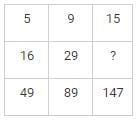Solution:

XY + Z = X(Y + Z)

⇒ XY + Z = XY + XZ

⇒ Z = XZ ⇒ Z - XZ = 0 ⇒ Z (1 - X) = 0

Either Z = 0 (in this case X can take any value) OR X = 1 (in this case Z can take any value).

X = 1 or Z = 0

5 × 3 + 1 = 16

16 × 3 + 1 = 49

9 × 3 + 2 = 29

29 × 3 + 2 = 89

15 × 3 + 3 = 48

48 × 3 + 3 = 147

QUESTION: 4

ARCHIPELAGO : ISLAND

Solution:

An ‘archipelago’ is ‘a group of islands’, i.e. it is it is formed of several ‘islands’. So the answer should be the option where the group of the latter word forms the former. Option 1 is incorrect as a group links forms a chain and not the other way round. Similarly, option C and D can be disqualified. An encyclopedia is ‘a book or set of books giving information on many subjects’. The correct answer is option B as many beads form a necklace.

*Answer can only contain numeric values
QUESTION: 5

There are three basket of fruits. First basket has twice the number of fruits in the second basket. Third basket has 3/4 th of the fruits in the first. The average of the fruits in all the basket is 30. The number of fruits in the first basket is

Solution:

Let the number of fruits in first basket is x

Fruits in 2nd basket = x/2

Fruits in 3rd basket = 3/4x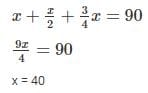QUESTION: 6

Until now only injectable vaccines against Swine Flu have been available. They have been primarily used by older adults who are at risk for complications from Swine Flu. A new vaccine administered in an oral form has proven effective in preventing Swine Flu in children. Since children are significantly more likely than adults to contract and spread Swine Flu, making the new vaccine widely available for children will greatly reduce the spread of Swine Flu across the population.

Which of the following, if true, most strengthens the argument?

Solution:

The passage points out that the oral form of vaccine will allow Swine Flu to be greatly reduced among the children who are more susceptible to catching the Flu (as compared to adults). Undoubtedly, the non painful method of oral vaccination is more likely to encourage the parents to get their children vaccinated who are skeptical of injections that cause pain. Thus, among all the given options, 4 strengthens the given argument. Options 1, 2 and 3 are completely irrelevant and 5 would weaken the argument as it cites the probable negative effects of the new vaccine which will definitely not encourage parents to get their children vaccinated.

QUESTION: 7

Four friends Rishabh, Keshav, Lavish and Hemang are out for shopping. Rishabh has less money than three times the amount that Keshav has. Lavish has more money than Keshav. Hemang has an amount equal to the difference of amounts with Keshav and Lavish. Rishabh has three times the money with Hemang. Each of them has to buy at least one shorts, or one sleeper, or one sleeveless t-shirt, or one goggle that is priced 200, 400, 600, and 1000 a piece, respectively. Lavish borrows 300 from Rishabh and buys a goggle. Keshav buys a Sleeveless t-shirt after borrowing 100 from Rishabh and is left with no money. Rishabh buys three shorts. What is the costliest item that Hemang could buy with his own money?

Solution:

Let, Rishabh has 'P' amount of money, Keshav has 'Q' amount of money, Lavish has 'R' amount of money, and Hemang has 'S' amount of money.

Therefore, according to the question

P < 3 × Q

R > Q,

S = R - Q

P = 3 × S

Also, Lavish borrows 300 from Rishabh and buys a goggle and Keshav buys a Sleeveless t-shirt after borrowing 100 from Rishabh and is left with no money. Implies that,

Q = 600 - 100 (Rishabh) = 500

R ≥ 1000 - 300 (Rishabh) ≥ 700

Also, Rishabh buys three shorts means he has a minimum of 1000 (100 + 300 + 3 × 200 = 1000) and the maximum is less than 3Q.

Therefore, 1000 ≤ P < 3Q

1000 ≤ P < 3 × 500

1000 ≤ P < 1500

Since Rishabh has three times the money with Hemang

1000 ≤ 3 × S  < 1500

333 ≤  S  < 500

Hence, Hemang could buy a sleeper with his own money.

QUESTION: 8

I wouldn’t _______ with a soldier who was wearing a metal _______, awarded for a display of _______.

The words that best fill the blanks in the above sentence are:

Solution:

The sentence implies that I would not poke a soldier who was wearing a metal medal which was awarded for the display of determination.

The meaning of the words is:

Meddle: interfere in something that is not one's concern

Medal: a metal disc typically of the size of a large coin and bearing an inscription or design, made to commemorate an event or awarded as a distinction to someone such as a soldier or athlete

Mettle: a person's ability to cope well with difficulties; spirit and resilience

Therefore, the correct sequence of words to fill in the blanks is option 2.

*Answer can only contain numeric values
QUESTION: 9

A paper sheet is in the shape of a right angle triangle and cut along a line parallel to hypotenuse leaving a smaller triangle. There was 25% reduction in the length of the hypotenuse of the triangle. If area of triangle initially was 28 cm2 then area of smaller triangle will be ______ cm2.

Solution: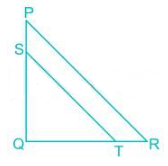Let PQR is the initial triangle and SQT is the final triangle.

ΔPQR is similar to ΔSQT

∵ ST = 0.75 PR

∴ SQ = 0.75 PQ

And QT = 0.75 QR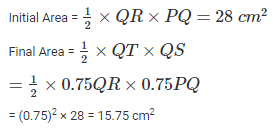QUESTION: 10

A drinks machine offers three selections - Tea, Coffee or Random but the machine has been wired up wrongly so that each button does not give what it claims. If each drink costs 50p, how much minimum money do you have to put into the machine to work out which button gives which selection?

Solution:

For the first drink press, the button labelled Random. We know this is NOT the Random button so if it dispenses Tea it is the Tea button likewise if it dispenses Coffee it is the Coffee button. From here it is simple to work out the other buttons knowing that they can't be what they say they are.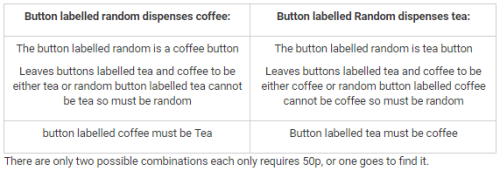*Answer can only contain numeric values
QUESTION: 11

Suffix of a string is any number of trailing symbols. What is the number of possible suffixes for the string of length 10?

Solution:

For a given string of length n, the number of possible suffixes = n+1

For example: In a string abcd, the suffixes are: ε, d, cd, bcd, abcd. Therefore, 5 suffixes are there in a string of length 4.

Hence, for a string of length 10, the number of suffixes is 11.

QUESTION: 12

If =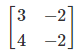,  find k so that A2=kA−2I where I is an identity matrix.

Solution: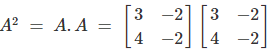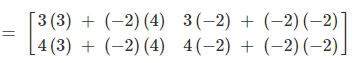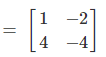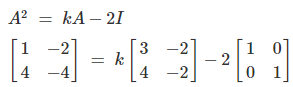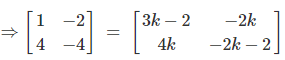As the two matrices are equal, their corresponding elements are also equal.

Comparing the corresponding elements:

4k = 4 ⇒ k = 1

QUESTION: 13

Consider sender’s sliding window size in Go - Back - n ARQ is equal to sender’s window size in Selective Repeat ARQ. Which of the following expression represents the relation between Go - Back - n and selective repeat ARQ in terms of n? (Where n is number of bits that are used to represent frame sequence number)

Solution:

Sender’s sliding window size in Go – Back – n ARQ = 2n – 1

Sender’s sliding window size in Selective Repeat ARQ = 2n-1

2n – 1 = 2n-1

2n – 2n-1 = 1

2n – 2n/2 = 1

2n+1 – 2n = 2

QUESTION: 14

Consider the schema a r(A, B, C, D, E) with the following functional dependencies:

A → BC

CD → E

B → D

E → A

The decomposition of the schema into r1(A, B, C) and r2(A, D, E) is _________.

Solution:

R1 and R2 form a lossless decomposition of R if at least one of the following functional dependencies is in F:

• R1 ∩ R→ R1

• R1 ∩ R2 → R2

In the given schema r(A, B, C, D, E), the candidate key is A because A+ = {A, B, C, D, E}

QUESTION: 15

Consider an array which stores a maximum of 100 elements. For case 1, the user inserts 1 element into an array. For case 2, the user inserts 100 elements into an array. What is the time complexity for both the cases?

Solution:

To store 1 element, 100 memory spaces are required.

To store 2 elements, 100 memory spaces are required.

.

.

.

To store n element, 100 memory spaces are required.

QUESTION: 16

Evaluate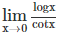Solution: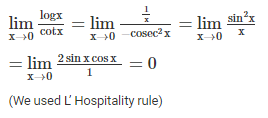QUESTION: 17

Which of the following expressions is valid for "There is an Indian who does not like watching Television" where

c(x): x likes watching television

Solution:

All Indians like watching Television: Ɐxc(x)

Negating the above expression

∼(Ɐxc(x))

Ǝx∼c(x)

QUESTION: 18

A frame consists of m data (i.e., message) bits and r redundant (i.e. check) bits. Column I consists of different type of codes and Column II consists of the way the redundant bits are calculated. Select the most appropriate option.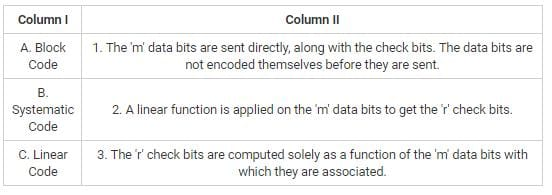Solution:

The correct answer is option 3 i.e. A-3, B-1, C-2.

In a block code, the r check bits are computed solely as a function of the m data bits with which they are associated, as though the m bits were looked up in a large table to find their corresponding r check bits.

In a systematic code, the m data bits are sent directly, along with the check bits, rather than being encoded themselves before they are sent.

In a linear code, the r check bits are computed as a linear function of the m data bits. Exclusive OR (XOR) or modulo 2 addition is a popular choice.

QUESTION: 19

Consider the following set of statements:

S1: If L1 and L2 are regular languages, then L1 - Lis also a regular language.

S2: Context free languages are closed under concatenation.

S3: Context free languages are closed under intersection.

S4: Regular language is closed under infinite union of regular languages.

Which of the given statements are true?

Solution:

Only statements S1 and S2 are true.

Context free languages are not closed under intersection. Also, regular languages are not closed under infinite union of regular languages.

QUESTION: 20

Mean of 12 observation was found to be 28. Later on, it was detected that an observation 62 was misread as 26. Then the correct mean of the observations is

Solution:

Given, mean of 12 observation was found to be 28.

Mean = sum of observations/number of observations.

Sum of observations = 12 × 28 = 336

Now, it was detected that an observation 62 was misread as 26.

∴ Correct mean =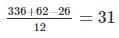QUESTION: 21

Which of the following activation record unit points to non-local data stored in other activation records?

Solution:

Temporary values: stores the values that arise in the evaluation of an expression.

Machine status: holds the information about the status of a machine just before the function call.

Access link: points to non-local data stored in other activation records.

Control link: points to activation record of a caller.

QUESTION: 22

Which of the following function is used to assign a name to a socket?

Solution:

bind is used to bind a name to socket.

When a socket is created with socket(2), it exists in a name space (address family) but has no address assigned to it. bind() assigns the address specified by addr to the socket referred to by the file descriptor sockfd. addrlen specifies the size, in bytes, of the address structure pointed to by addr.

*Answer can only contain numeric values
QUESTION: 23

Let A = {1,2,3,4}. What is the number of irreflexive relations which can be formed on the set A?

Solution:

Note: The number of irreflexive relations on set A when |A| = n is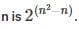Here |A| = 4

Therefore, total number of irreflexive relations =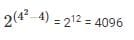QUESTION: 24

Consider an interpolation search which is an improvement over binary search where the values in a sorted array are uniformly distributed. In interpolation search construction of new data points take place at different locations according to the value of the key being searched. Find the time complexity of interpolation search.

Solution:

To narrow down the search space binary search uses mid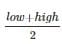while interpolation search uses mid =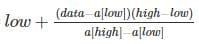On average the interpolation search makes about log (log n) comparisons if the elements are uniformly distributed, where n is the number of elements to be searched.

*Answer can only contain numeric values
QUESTION: 25

A group consists of equal number of men and women. Of this group 20% of the men and 50% of the women are unemployed. If a person is selected at random from this group, the probability of the selected person being employed is _______.

Solution:

M for men, W for women, E for employed & U for unemployed

P(M) = 0.5, P(W) = 0.5

P(U/M) = 0.2 & P(U/W) = 0.5

∴ Total probability is

P(U) = P(M). P(U/M) + P(W) ⋅ P(U/W)

⇒ P(U) = 0.35

∴ P(E) = 1 – P(U) = 1-0.35 = 0.65

*Answer can only contain numeric values
QUESTION: 26

There are 17 engineers and 3 official languages. Every pair of engineers communicates in one of the official languages. What is the number of engineers communicating in the same language pairwise?

Solution:

Since we need to find the number of engineers communicating in the same language pairwise, the number of pairs that can be formed = 9.

By pigeonhole principle, there are ceil (9/3)  = 3  ​engineers communicating in the same language pairwise.

QUESTION: 27

Which of the following statement is correct about WiFi?

I. WiFi systems are full duplex.

II. WiFi uses access control protocol - CSMA/CA.

III. Channel bandwidth of high rate wifi is 25 MHz

Solution:

IEEE 802.11 wireless LANs use a media access control protocol called Carrier Sense Multiple Access with Collision Avoidance (CSMA/CA).

All WiFi networks are contention-based TDD systems, where the access point and the mobile stations all use the same channel. Because of the shared media operation, all WiFi networks are half duplex.

QUESTION: 28

Which of the following indexes is created on a non-key field and defined on an ordered data file?

Solution:

The correct answer is option 1 i.e. clustering index.

A clustering index is defined on an ordered data file. The data file is ordered on a non-key field.

The primary index is defined on an ordered data file. The data file is ordered on a primary key field.

A secondary index is defined on an unordered data file. It can be either a candidate key or some non-key field.

*Answer can only contain numeric values
QUESTION: 29

Consider the following languages over the input alphabet {a,b}:

A: {ambn | m>n}

B: {xcy | x,y Є (a,b)*}

C: {aibj | i = 2j}

D: {wwwR | w Є (a,b)*}

The number of context free languages is ____.

Solution:

Out of the given languages, only D i.e. {wwwR | w Є (a,b)*} is not a context free language.

Push down automata for all the other languages can be drawn.

QUESTION: 30

Consider a simple paging system with 1024 MB of physical memory and the page size of 4KB with a logical address space of 128 pages. Find the number of bits used to represent physical address, frame number and page number.

Solution:

Physical memory = 1024 MB = 1024 × 1024 × 1024 bytes = 210 × 210 × 210 = 230

Hence, 30 bits are required to represent physical address.

Page size = 4 KB = 4 × 210 = 212

Number of frames =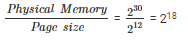Hence, 18 bits are required to represent frame number.

128 pages i.e. 27 pages being accessed. Hence, 7 bit represent page number.

QUESTION: 31

Apply the Dijkstra’s shortest path algorithm on the following graph.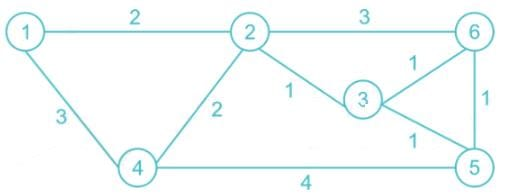The correct path from vertex 1 to 6 is ______.

Solution:
QUESTION: 32

Consider the 4 to 1 multiplexer with two select lines S1 and S0 given below.

The minimal sum of products form of the Boolean expression for the output F of the multiplexer is

Solution:

The correct answer is option 2.

The output is given by:

F = P'Q' + P'QR + PQR'

= P'[Q' + QR] + PQR'

= P'[Q' + Q][Q' + R] + PQR'

= P'[Q' + R] + PQR'

= P'Q' + P'R + PQR'

*Answer can only contain numeric values
QUESTION: 33

Consider a disk system with 100 cylinders. The requests to access the cylinders occur in the following sequence:

16, 24, 7, 9, 25, 11, 8, 87

Assuming that the head is currently at cylinder 58, what is the time taken (in ms) to satisfy all requests if first come first serve policy is used?

Solution:

(58 – 16) + (24 - 16) + (24 - 7) + (9 - 7) + (25 - 9) + (25 - 11) + (11 - 8) + (87 - 8)

= 42 + 8 + 17 + 2 + 16 + 14 + 3 + 79

= 181 ms

QUESTION: 34

Which of the following instruction cannot be represented in 1 - address instruction format?

Solution:

LOAD and STORE are 1 - address instruction. LOAD is used to load data from memory to accumulator. STORE is used to store the accumulator result in memory.

e.g. LOAD X   AC = M[X]

e.g. STORE YM[Y] = AC​

MOV is a 2 – address instruction. MOVE is used to move contents of a register to memory and vice versa or from one register to another.

e.g. MOV R1, X          R1 = M[X]

QUESTION: 35

Let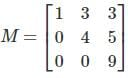then

Solution:The given matrix is upper triangular matrix. Hence Eigen values = Diagonal elements = 1,4, 9

M has distinct Eigen values, hence eigen vectors are linearly independent.

So, M is diagonalizable

The eigen values of M2 = 12, 42, 92

= 1,76,25

M2 also having distinct eigen values.

Hence both M and M2 are diagonalizable.

QUESTION: 36

Which of the control signal specifies that the ALU operation should be determined from the function bits?

Solution:

Control signal ALUOp determines either specifies the ALU operation to be performed or specifies that the operation should be determined from the function bits.

*Answer can only contain numeric values
QUESTION: 37

Consider a multicore system in which an application has 40% serial component and 60% parallel component. After executing this application on a system with three processing cores, the maximum potential gain achieved is ________. (Calculate value up to two decimal places)

Solution:

In parallel computing, Amdahl's law is mainly used to predict the theoretical maximum speed-up for program processing using multiple processors.

If S is the portion of the application that must be performed serially on a system with N processing cores, the formula appears as follows: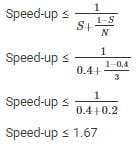*Answer can only contain numeric values
QUESTION: 38

Consider a LAN with five nodes. Time is divided into fixed-size slots. A node can begin its transmission only at the beginning of a slot. A collision occurs if more than two nodes transmits in the same slot. The probabilities of generation of a frame in a time slot by these LAN are 0.2, 0.3, 0.25, 0.16 and 0.09, respectively. The probability of transmission in the first slot without any collision by any of these five stations is _________. (Compute the answer up to 2 decimal places.)

Solution:

Probability of transmission on the first slot by 1st node:

= 0.2 × (1 – 0.3) × (1 – 0.25) × (1 – 0.16) × (1 – 0.09)

= 0.2 × 0.7 × 0. 75 × 0.84 × 0.91

Probability of transmission on the first slot by 2nd node:

= (1 - 0.2) × 0.3 × (1 – 0.25) × (1 – 0.16) × (1 – 0.09)

= 0.8 × 0.3 × 0. 75 × 0.84 × 0.91

Probability of transmission on the first slot by 3rd node:

= (1 - 0.2) × (1 - 0.3) × 0.25 × (1 – 0.16) × (1 – 0.09)

= 0.8 × 0.7 × 0.25 × 0.84 × 0.91

Probability of transmission on the first slot by 4th node:

= (1 - 0.2) × (1 - 0.3) × (1 - 0.25) × 0.16 × (1 – 0.09)

= 0.8 × 0.7 × 0.75 × 0.16 × 0.91

Probability of transmission on the first slot by 5th node:

= (1 - 0.2) × (1 - 0.3) × (1 - 0.25) × (1 - 0.16) × 0.09

= 0.8 × 0.7 × 0.75 × 0.84 × 0.09

Probability = 0.080262 + 0.137592 + 0.107016 + 0.061152 + 0.031752 = 0.4177

*Answer can only contain numeric values
QUESTION: 39

Consider the DFA given below. What is the number of states obtained after minimizing the given DFA?

Solution:

Since the state q2 is not reachable from any of the states, we do not consider it in the transition table. The  modified transition diagram is as follows:

0 equi sets: [q0, q1, q3], [q4]

1 equi sets: [q0], [q1, q3], [q4]

2 equi sets: [q0], [q1, q3]. [q4]

Therefore, the number of states in minimized DFA is 3.

*Answer can only contain numeric values
QUESTION: 40

An operating system uses Shortest Job first (SJF) process scheduling algorithm. Consider the arrival times and execution times (in milliseconds) for the following processes: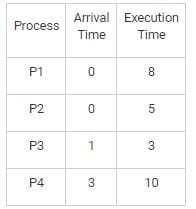What is the average response time (in milliseconds)?

Solution:

Gantt chart: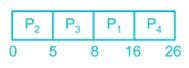Response time is the amount of time from when a request was submitted until the first response is produced.

Response time = submission time – Arrival time

Response time of process P1 = 8 – 0 = 8

Response time of process P2 = 0 – 0 = 0

Response time of process P3 = 5 – 1 = 4

Response time of process P4 = 16 – 3 = 13

Average response time =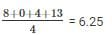QUESTION: 41

Consider an unrolled linked list with n elements. This list stores multiple elements in each node. What is the worst case time complexity to find the kth element if the number of nodes and the number of elements in each node are equal?

Solution:

An unrolled linked list stores multiple elements in each node.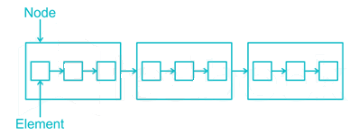Here, the number of elements in each node is equal to the number of nodes in a list. Hence, there are total √n elements in each node and total √n  nodes in a list.

To find the kth element, traverse the list up to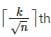node. If an element is present in the last node, total  √n nodes should be traversed. Hence, time complexity to search an element is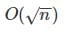QUESTION: 42

Consider the following query:

select distinct emp_id

from employee

where project = ’Alpha’ and joining_year = 2009 and

emp_id not in (select emp_id

from employee

where project= ’Beta’ and joining_year= 2009);

What does the given query perform?

Solution:
*Answer can only contain numeric values
QUESTION: 43

Frames of 20 kb are sent over a 10 Mbps duplex link between 2 hosts. Propogation time is 45 ms. Suppose that the sliding window protocol is used with the sender window size of 16 and acknowledgments are always piggybacked. After sending 35 frames, what is the minimum time the sender will have to wait before starting transmission of the next frame?

Solution:

Transmission time (Tx) for 1 frame = L/B

= 20 × 10^3/ 10 × 10^6

= 2 ms

Transmission time for 35 frame = 70 ms

Round trip time = 90 ms

The sender cannot receive acknowledgement before 90 ms.

After sending 35 frames, the minimum time the sender will have to wait before starting transmission of the next frame = 90 – 70 = 20 ms

QUESTION: 44

What is the generating function for the sequence <2, 0, 2, 0, 2, 0...>?

Solution:

The generating function for the sequence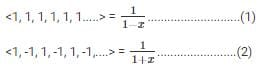Adding (1) and (2)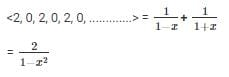QUESTION: 45

The expression (P ⇒ Q) ˄ (R ⇒ Q) is equivalent to

Solution:

The correct answer is option 2 i.e. (P ˅ R) ⇒ Q.

The truth table for the given expressions is: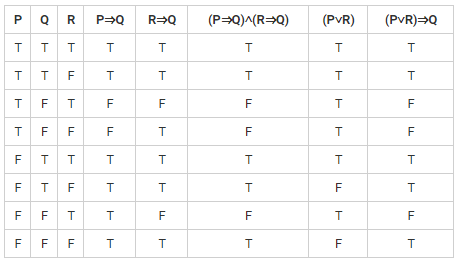*Answer can only contain numeric values
QUESTION: 46

Consider six memory partitions of size 200 KB, 400 KB, 600 KB, 500 KB, 300 KB, and 250 KB, where KB refers to kilobyte. These partitions need to be allotted to four processes of sizes 357 KB, 210 KB, 468 KB and 491 KB in that order. If the best fit algorithm is used, which partitions are NOT allotted to any process?

Solution:
QUESTION: 47

What is the output of the following code?

int main()

{

float k = 5.375;

char *m;

int i;

m = (char*)&k;

for(4; 7; 2)

printf("%d ", m);

return 0;

}

Solution:

m is similar to some address which returns some garbage value. Here in condition of for loop, there is a non-zero value, thus it becomes infinite for loop.

*Answer can only contain numeric values
QUESTION: 48

The keys 25, 78, 15, 109, 50, and 6 are inserted into an initially empty hash table of length 7 using quadratic probing with hash function h(k)= k mod 7. Find the maximum number of iteration (i) to solve collision.

Solution:

If slot hash(x) % S is full, then use (hash(x) + 1*1) % S for next iteration use (hash(x) + 2*2)

For ith iteration use (hash(x) + i*i)

h(25) = 25 mod 7 = 4

h(78) = 78 mod 7 = 1

h(15) = 15 mod 7 = 1 → 15 + 1 × 1 mod 7 = 2

h(109) = 109 mod 7 = 4 → 109 + 1 × 1 mod 7 = 5

h(50) = 50 mod 7 = 1 → 50 + 1 × 1 mod 7 = 2 → 50 + 2 × 2 mod 7 = 5 → 50 + 3 × 3 mod 7 = 3

h(6) = 6 mod 7 = 6

QUESTION: 49

Classless Inter-domain Routing (CIDR) receives a packet with address 182.25.110.68. The router’s routing table has the following entries: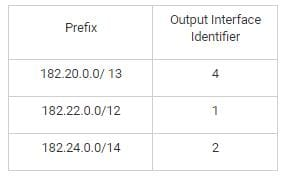The identifier of the output interface on which this packet will be forwarded is ______.

Solution:

182.25.110.68 -> 182.00011001.110.68

182.20.0.0/ 13 -> 182.00011000.0.0

Bitwise AND result -> 182.00011000.0.0 -> 182.24.0.0

182.25.110.68 -> 182.00011001.110.68

182.22.0.0/ 12 -> 182.00010000.0.0

Bitwise AND result -> 182.00010000.0.0 -> 182.16.0.0

182.25.110.68 -> 182.00011001.110.68

182.24.0.0/ 14 -> 182.00011000.0.0

Bitwise AND result -> 182.00011000.0.0 -> 182.24.0.0

QUESTION: 50

Let 'i' represents the cache line number, 'j' represents main memory block number and 'm' represents the number of lines in the cache. Which of the following is the correct expression for direct mapping?

Solution:

Direct mapping maps each block of main memory into only one possible cache line. The mapping is expressed as:

i = j modulo m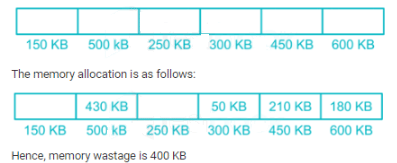*Answer can only contain numeric values
QUESTION: 51

Consider the grammar with the following translation rules and E as the start symbol.

E -> E @ T                 E1.trans = E2.trans + T.trans

E -> T                        E.trans  = T.trans

T -> T \$ F                  T1.trans = T2.trans * F.trans

T -> F                        T.trans  = F.trans

F -> INTLITERAL       F.trans  = INTLITERAL.value

F -> (E)                       F.trans  = E.trans​

Compute E.value for the root of the parse tree for the expression:4 \$ (7 @ 8)

Solution:

Syntax-directed translation refers to a method of compiler implementation where the source language translation is completely driven by the parser.

A common method of syntax-directed translation is translating a string into a sequence of actions by attaching one such action to each rule of a grammar.

4 \$ (7 @ 8)

4 *(7+8)

4*15

60

QUESTION: 52

Consider the following infix expression. While converting this infix expression to postfix expression, what will be the stack content (from bottom to top) after scanning operand 5?

((13 × 4) + 16) ÷ 4 – ((8 × 5) ÷ 10)

Solution: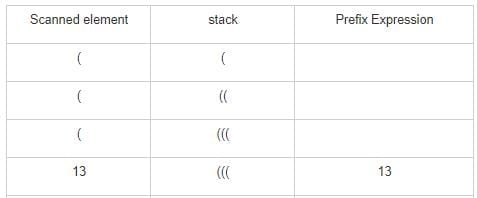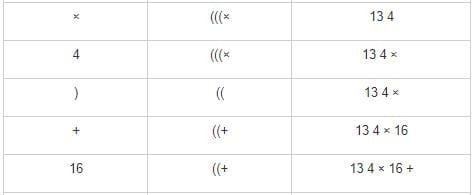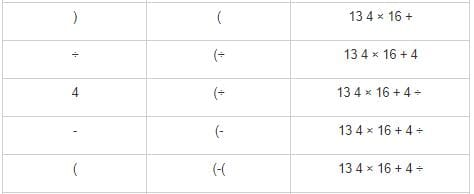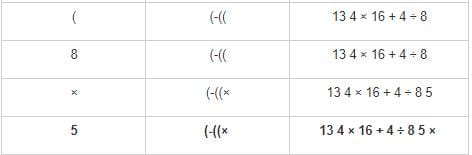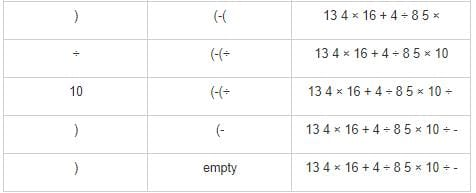QUESTION: 53

Consider the following C-fragment:

#include<stdio.h>

int (*foo())

{

int a = {1,2,3};

printf("%d", *a);

return &a;

}

int main()

{

int (*p);

p = foo();

printf("%d", *(*p + 2));

return 0;

}

What is the output of the given program?

Solution:

The function foo() holds an array which returns the address of the first element of the array a.

Also, p holds the address of the first element of the array a. Thus, *p returns the address of the first element of the array a and **p returns the value of the first element of the array a.

Therefore, (*p + 2) will point to the third location of the array. *(*p+2) will now return the value of the third element of the array.

Hence, the output will be 13.

QUESTION: 54

Consider the following schedules: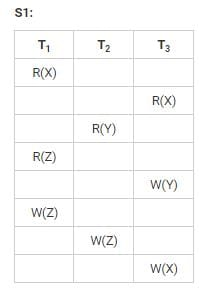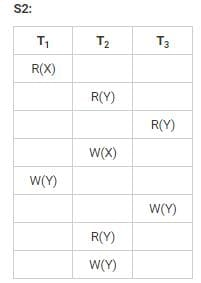Which of the given schedules is/are conflict serializable?

Solution:

The wait-for-graph for the given schedules is as follows:

Since there is no cycle in S1, S1 is conflict serializable.

QUESTION: 55

In HTTP request message, head of the request is consist of

Solution:

A start-line describes the requests to be implemented, or its status of whether successful or a failure.

An optional set of HTTP headers specifies the request, or describes the body included in the message.

The start-line and HTTP headers of the HTTP message are collectively known as the head of the requests, whereas its payload is known as the body.

*Answer can only contain numeric values
QUESTION: 56

What is the number of perfect matching in a complete graph K6?

Solution:

The number of perfect matching in a complete graph K2n is given by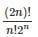Therefore, the number of perfect matching in a complete graph K6 is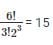*Answer can only contain numeric values
QUESTION: 57

Consider the following strings:

S1 = PQPTSR

S2 = QPRQPS

Find the length of the longest common subsequence by using dynamic programming.

Solution:
QUESTION: 58

What is the output of the function fun(-1+2419)?

int fun(int n)

{

if (n == 0)

return 0;

int temp = n % 10 + fun(n / 10);

if(temp>9)

return fun(temp);

else

return temp;

}

Solution:

fun(-1+2419) → fun(2418)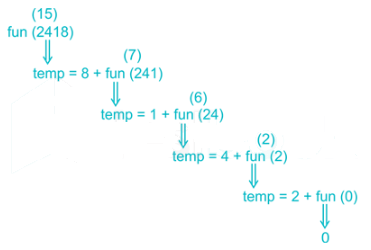fun(2418) returns value 15 which is greater than 9. Hence it will call fun(15)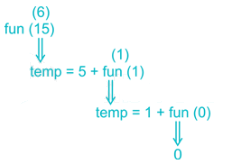QUESTION: 59

Which of the following is the drawbacks of the I/O mode of transfer?

Solution:

The disadvantages of I/O mode of transfer are:

1. The I/O transfer rate is limited by the speed with which the processor can test and service a device.
2. The processor is tied up in managing an I/O transfer; a number of instructions must be executed for each I/O transfer.

Programmed I/O is simple to implement and does not require any special software or hardware.

Programmed I/O is probably the most common I/O technique because it is very cheap and easy to implement, and in general does not introduce any unforeseen hazards.

*Answer can only contain numeric values
QUESTION: 60

The minimum positive integer p such that 8Y (mod 23) = 1 then Y is ______.

Solution:

Fermat's little theorem states that if p is a prime number, then for any integer a, the number ap − a is an integer multiple of p. In the notation of modular arithmetic, this is expressed as ap ≡ a (mod p)

If a is not divisible by p, Fermat's little theorem is equivalent to

ap-1 ≡ 1 (mod p)

Here a = 8 and p = 23

Y = 23 – 1 = 22

*Answer can only contain numeric values
QUESTION: 61

Consider the following grammar:

S0-> AS|PB|SB|S

S → AS|QB|SB

A → RS|XS|a

B → TS|VV|US|XS|a

X → a

Y → b

V → b

P → AS

Q → a

R → XA

T → SY

U → XA

What is the number of steps required to derive aaaaaaaa?

Solution:

The above grammar is in CNF.

Therefore, the number of steps required in the derivation of an n-length string from the given CNF is 2n-1.

'aaaaaaaa' is a string of lenght 8, therefore, to derive it, the number of steps required = 15.

Note: It can be easily proved by derivating the string from the given grammar.

*Answer can only contain numeric values
QUESTION: 62

Consider the following equation:

(247)8 = (1132)x

What will be the value of 'x' so that the equation is satisfied?

Solution:

On converting (247)8 into binary, we get (010100111)2.

(247)= (010100111)2 = (167)10

(167)10 = (1132)5

Therefore, x = 5

QUESTION: 63

Consider the following recursive function:

int sum(n int)

{

if n == 1

{

return 1

}

return sum(n) + Sum(n-1)

}

What is the time complexity of the above code?

Solution:

Recurrence relation for above code is

T(1) = 1

T(n) = 1 + T(n-1), when n > 1

1 + T(n-1)

= 1 + (1 + T(n-2))

= 2 + T(n-2)

= 2 + (1 + T(n-3))

= 3 + T(n-3) …

= k + T(n-k) …

= n - 1 + T(1)

= n - 1 + 1

= Θ(n)

QUESTION: 64

A typical SQL query has the form

select A1, A2,..., An

from r1, r2,...,rm

where P;

Each Ai represents an attribute, and each ri a relation. P is a predicate.

What is the relational algebra expression for the given query?

Solution:

The correct answer is option 3 i.e.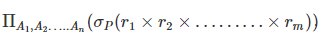The select operation is represented by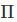while the project operation is represented by σ.

QUESTION: 65

Consider the following grammar:

S → AB

B → +S

B → ε

A → int C

A → (S)

C → *A

C → ε

Find the follow of A.

Solution:

First(S) = {int, (}

First(A) = {int, (}

First(B) = { ε, +}

First(C) = { ε, *}​

Follow(S) = {), \$}

Follow (A) = {), \$, +}

Follow (B) = { ), \$}

Follow (C) = {), \$, +}Use Code STAYHOME200 and get INR 200 additional OFF Use Coupon Code